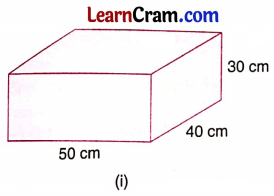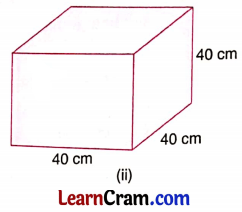# DAV Class 8 Maths Chapter 14 Worksheet 3 Solutions

The DAV Maths Book Class 8 Solutions Pdf and DAV Class 8 Maths Chapter 14 Worksheet 3 Solutions of Mensuration offer comprehensive answers to textbook questions.

## DAV Class 8 Maths Ch 14 Worksheet 3 Solutions

Question 1.
Find the length of the side of a cube whose total surface area measures 600 cm2.
Solution:
Let the length of the cube be l cm
∴ The total surface area of the cube = 6l2
⇒ 600 = 6l2
⇒ l2 = 100
⇒ l = 10 cm
Hence, the length of the side of the cube is 10 cm.Question 2.
The length, breadth, and height of a room are 5 m, 4 m, and 3 m respectively. Find the cost of whitewashing the inner of the room and ceiling at the rate of ₹ 50 per square meter.
Solution:
Here l = 5 m, 6 = 4 m and h = 3 m
∴ Area of the walls and the ceiling to be whitewashed = area of the 4 walls + area of the ceiling
= 2[bh + lh] + lb
= 2[4 × 3 + 5 × 3] + 5 × 4
= 2[12 + 15] + 20
= 54 + 20
= 74 m2
The cost of whitewashing = 74 × 50 = ₹ 3700.

Question 3.
Find the total surface area of a closed cardboard box of length 0.5 m, breadth 25 cm, and height 15 cm.
Solution:
Here l = 0.5 m = 50 cm, b = 25 cm and h = 15 cm
∴ T.S.A. = 2[lb + bh + lh]
= 2[50 × 25 + 25 × 15 + 50 × 15]
= 2[1250 + 375 + 750]
= 2
= 4750 cm2
Hence, T.S.A. = 4750 cm2.Question 4.
You are given two boxes. Which box will need more paper to cover the whole box?Solution:
(i) Total surface of area of the cuboid = 2[lb + bh + lh]
= 2[50 × 40 + 40 × 30 + 50 × 30]
= 2[2000 + 1200 + 1500]
= 2
= 9400 cm2

(ii) Total surface area of the cube = 6l2
= 6 × (40)2
= 6 × 40 × 40
= 9600 cm2
Hence, (ii) box will need more paper to cover.

Question 5.
The dimensions of an oil tin are 26 cm × 26 cm × 45 cm. Find the area of tin sheet required to make 20 such tins.
Solution:
Here l = 26 cm, b = 26 cm and h = 45 cm
∴ T.S.A. of the tin sheet = 2[lb + bh + lh]
= 2126 × 26 + 26 × 45 + 26 × 45]
= 2[676 + 1170 + 1170]
= 2
= 6032 cm2
∴ The area of the tin sheet to make 20 such tins = 6032 × 20 = 120640 cm2 = 12.064 m2.

Question 6.
A swimming pool is 20 m in length, 15 m in breadth, and 4 m in depth. Find the cost of cementing its floor and walls at ₹ 35 per m2.
Solution:
Area of the walls and floor = 2 [lh + bh] + lb
= 2[20 × 4 + 15 × 4] + 20 × 15
= 2[80 + 60] + 300
= 2 × 140 + 300
= 280 + 300
= 580 m2
∴ The cost of cementing = 580 × 35 = ₹ 20300.Question 7.
A cubical box with a lid has a length of 30 cm. Find the cost of painting the inside and outside of the box at ₹ 5.50 of per m2.
Solution:
The total surface area of the cubical box outside and inner side = 2 × 6l2
= 2 × 6 × 30 × 30
= 10800 cm2
= 1.08 m2
∴ The cost of painting the box on both sides = ₹ 5.50 × 1.08 = ₹ 5.94.

Question 8.
Two cubes of side 4 cm are fixed together. Find the total surface area of the new solid formed.
Solution:
When the two cubes are fixed together the new solid formed will be a cuboid whose length = 4 + 4 = 8 cm